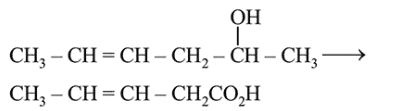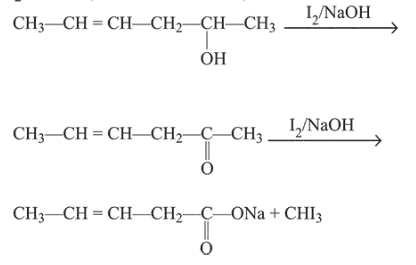# Which is the most suitable reagent for the following transformation?

Question:

Which is the most suitable reagent for the following transformation?1. Tollen's reagent

2. $\mathrm{I}_{2} / \mathrm{NaOH}$

3. $\mathrm{CrO}_{2} \mathrm{Cl}_{2} / \mathrm{CS}_{2}$

4. alkaline $\mathrm{KMnO}_{4}$

Correct Option: , 2

Solution:

The most suitable reagent for the given reaction is $\mathrm{I}_{2} / \mathrm{NaOH}$ (Iodoform reaction).